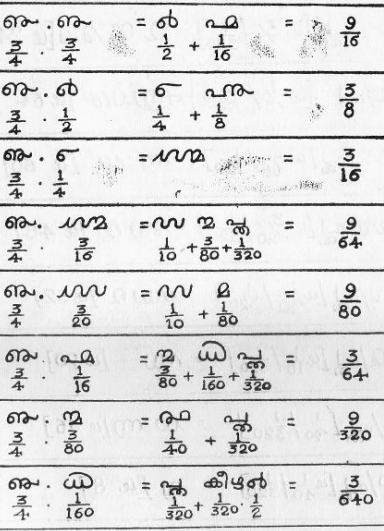## Monday 7 February 2022

### Unicode Trivia U+0D5A

Codepoint: U+0D5A "MALAYALAM FRACTION THREE EIGHTIETHS"
Block: U+0D00..0D7F "Malayalam"

Malayalam script is the main method of writing the Malayalam language of South West India, spoken by about forty million people. It is a Brahmic script, imported into Unicode 1.0 along with the other scripts covered by ISCII 1991.

Although "Malayalam" is a palindrome, I want to talk about their fractions. I like Unicode fractions. Have you noticed?

The original Unicode 1.0 block didn't have any had Malayalam-specific fraction codepoints. These were added in Unicode 6.0 (proposal N2970 by V. S. Umamaheswaran 2005-08-23) and Unicode 9.0 (proposal N4429 by Shriramana Sharma 2013-04-25). The latter proposal, N4429, gives details of the old system of Malayalam fractions used before decimalisation:Malayalam Fraction Multiplication ExamplesPrācīna Gaṇitaṃ Malayāḷattil, Prof C K Moosathu, Kerala State Institute of Language, 1980

It appears to be similar to Tamil fractions, using 320 as a common denominator:

• 1/320 (one three-hundred-and-twentieth) = U+0D2A U+0D4D U+0D24 = "പ്ത" (muntiri)
• 2/320 (one one-hundred-and-sixtieth) = U+0D58 = "൘" (arakkāṇi)
• 4/320 (one eightieth) = U+0D2E = "മ" (kāṇi)
• 8/320 (one fortieth) = U+0D59 = "൙" (aramā)
• 12/320 (three eightieths) = U+0D5A = "൚" (mūnnukāṇi)
• 16/320 (one twentieth) = U+0D5B = "൛" (orumā)
• 20/320 (one sixteenth) = U+0D76 = "൶" (mākāṇi)
• 32/320 (one tenth) = U+0D5C = "൜" (raṇṭumā)
• 40/320 (one eighth) = U+0D77 = "൷" (arakkāl)
• 48/320 (three twentieths) = U+0D5D = "൝" (mūnnumā)
• 60/320 (three sixteenths) = U+0D78 = "൸" (muṇṭāṇi)
• 64/320 (one fifth) = U+0D5E = "൞" (nālŭmā)
• 80/320 (one quarter) = U+0D73 = "൳" (kāl)
• 160/320 (one half) = U+0D74 = "൴" (ara)
• 240/320 (three quarters) = U+0D75 = "൵" (mukkāl)

Note that there's no single codepoint for "1/320" (the sequence U+0D2A U+0D4D U+0D24 achieves the required glyph) and "4/320" shares a glyph with U+0D2E "MALAYALAM LETTER MA". Other than these two exceptions, the names of the fraction codepoints are as expected:

• U+0D58 "MALAYALAM FRACTION ONE ONE-HUNDRED-AND-SIXTIETH"
• U+0D59 "MALAYALAM FRACTION ONE FORTIETH"
• U+0D5A "MALAYALAM FRACTION THREE EIGHTIETHS"
• U+0D5B "MALAYALAM FRACTION ONE TWENTIETH"
• U+0D5C "MALAYALAM FRACTION ONE TENTH"
• U+0D5D "MALAYALAM FRACTION THREE TWENTIETHS"
• U+0D5E "MALAYALAM FRACTION ONE FIFTH"
• U+0D73 "MALAYALAM FRACTION ONE QUARTER"
• U+0D74 "MALAYALAM FRACTION ONE HALF"
• U+0D75 "MALAYALAM FRACTION THREE QUARTERS"
• U+0D76 "MALAYALAM FRACTION ONE SIXTEENTH"
• U+0D77 "MALAYALAM FRACTION ONE EIGHTH"
• U+0D78 "MALAYALAM FRACTION THREE SIXTEENTHS"

All fractions with a denominator of 320 can easily be represented by adding together parts. In the image above, the third answer is:

3/16 = 60/320 = "൸" (U+0D78)

3/64 = (12 + 2 + 1)/320 = "൚൘പ്ത" (U+0D5A U+0D58 U+0D2A U+0D4D U+0D24)

There's the possibility of ambiguity here because we could construct 3/64 using (12+2+1)/320 or (8+4+2+1)/320, but I assume you always pick the biggest part you can at every step.

Many Indic systems use base-4 for fractions, so the choice of 320 as the common denominator seems peculiar to me. If it was based on a currency or metric with subdivisions of 320, I haven't been able to find any references to that. I suppose base-320 has an advantage over dyadic fractions in that it is divisible by 5, 10, etc. But why not choose base-60 like the Sumerians, or even base-360?

• Factors of 320: 1, 2, 4, 5, 8, 10, 16, 20, 32, 40, 64, 80, 160, 320 (14)
• Factors of 360: 1, 2, 3, 4, 5, 6, 8, 9, 10, 12, 15, 18, 20, 24, 30, 36, 40, 45, 60, 72, 90, 120, 180, 360 (24)

I assume that the Malayali preferred powers of two over the convenience of dividing into thirds and the like. But the choice of 320 means that the sequence breaks down if you double in size from the smallest:

• 1/320 (one three-hundred-and-twentieth) = U+0D2A U+0D4D U+0D24 = "പ്ത" (muntiri)
• 2/320 (one one-hundred-and-sixtieth) = U+0D58 = "൘" (arakkāṇi)
• 4/320 (one eightieth) = U+0D2E = "മ" (kāṇi)
• 8/320 (one fortieth) = U+0D59 = "൙" (aramā)
• 16/320 (one twentieth) = U+0D5B = "൛" (orumā)
• 32/320 (one tenth) = U+0D5C = "൜" (raṇṭumā)
• 64/320 (one fifth) = U+0D5E = "൞" (nālŭmā)
• What now?

Or if you halve in size:

• 160/320 (one half) = U+0D74 = "൴" (ara)
• 80/320 (one quarter) = U+0D73 = "൳" (kāl)
• 40/320 (one eighth) = U+0D77 = "൷" (arakkāl)
• 20/320 (one sixteenth) = U+0D76 = "൶" (mākāṇi)
• 10/320 (one thirty-second) = missing
• 5/320 (one sixty-fourth) = missing
• What now?
Or if you quarter in size (base-4 fractions):
• 80/320 (one quarter) = U+0D73 = "൳" (kāl)
• 160/320 (one half) = U+0D74 = "൴" (ara)
• 240/320 (three quarters) = U+0D75 = "൵" (mukkāl)

• 20/320 (one sixteenth) = U+0D76 = "൶" (mākāṇi)
• 40/320 (one eighth) = U+0D77 = "൷" (arakkāl)
• 60/320 (three sixteenths) = U+0D78 = "൸" (muṇṭāṇi)

• 5/320 (one sixty-fourth) = missing
• 10/320 (one thirty-second) = missing
• 15/320 (three sixty-fourths) = missing

• What now?

The remaining fractions (if you remove those found in the three schemes immediately above) are:

• 12/320 (three eightieths) = U+0D5A = "൚" (mūnnukāṇi)
• 48/320 (three twentieths) = U+0D5D = "൝" (mūnnumā)

These suggest division into fifths and then quartering thereafter:

• 64/320 (one fifth) = U+0D5E = "൞" (nālŭmā)

• 16/320 (one twentieth) = U+0D5B = "൛" (orumā)
• 32/320 (one tenth) = U+0D5C = "൜" (raṇṭumā)
• 48/320 (three twentieths) = U+0D5D = "൝" (mūnnumā)

• 4/320 (one eightieth) = U+0D2E = "മ" (kāṇi)
• 8/320 (one fortieth) = U+0D59 = "൙" (aramā)
• 12/320 (three eightieths) = U+0D5A = "൚" (mūnnukāṇi)

• 1/320 (one three-hundred-and-twentieth) = U+0D2A U+0D4D U+0D24 = "പ്ത" (muntiri)
• 2/320 (one one-hundred-and-sixtieth) = U+0D58 = "൘" (arakkāṇi)
• 3/320 (three three-hundred-and-twentieths) = missing
That's the only way I can think that they'd bother to have a symbol that became U+0D5A "MALAYALAM FRACTION THREE EIGHTIETHS"